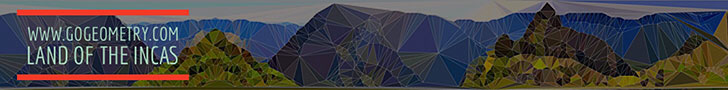Geometry Problems 611-620: Triangle, Parallelogram, Rectangle, Incircle, Congruence# Geometry Problems 611 - 620Geometry Problem 611
Altitude of a Triangle, Perpendicular, Collinear Points, Parallel.

Geometry Problem 612
Altitude of a Triangle, Perpendicular, Congruence.

Geometry Problem 613
Altitude of a Triangle, Perpendicular, Collinear Points.

Geometry Problem 614
Parallelogram, Diagonals, Intersecting Lines, Parallel Lines.

Geometry Problem 615
Right Triangle with a Square, Diagonals, Center, Area of Quadrilateral.

Geometry Problem 616
Parallelogram, Diagonals, Intersecting Lines, Parallel Lines.

Geometry Problem 617
Right Triangle Area, Incircle, Incenter, Tangent, 90 Degrees.

Geometry Problem 618
Triangle Area, 60 Degrees, Incircle, Incenter, Tangent.

Geometry Problem 619
Triangle Area, 30 Degrees, Incircle, Incenter, Tangent.

Geometry Problem 620
Three Rectangles, Diagonals, Centers, Angles.

 Home | Sitemap | Search | Geometry | Problems | Visual Index | 10 Problems | Email | Post a comment | By Antonio Gutierrez